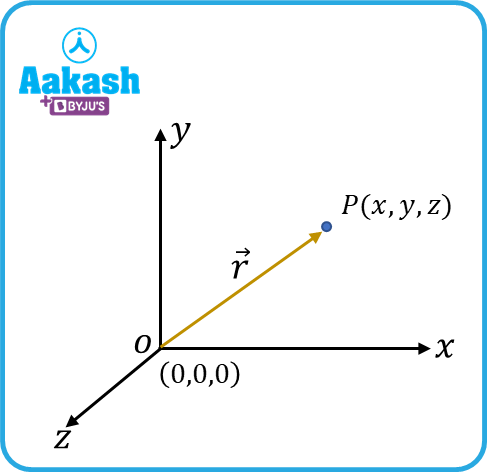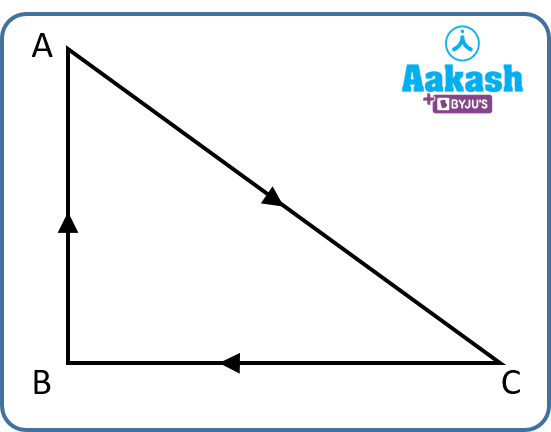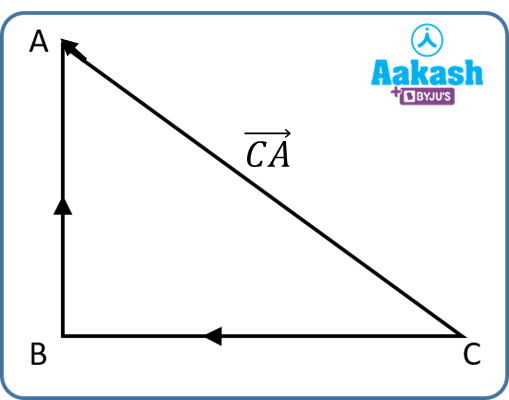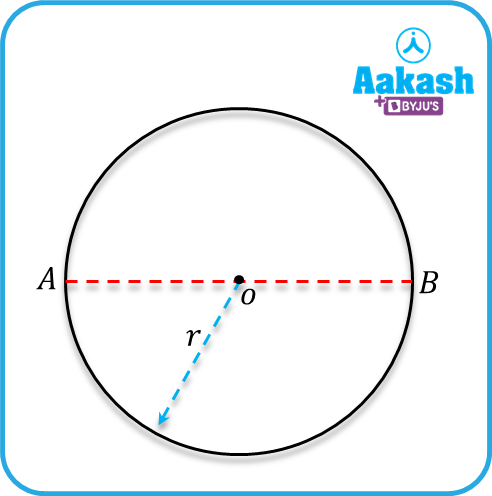• Call Now

1800-102-2727•

# Difference between Distance and Displacement - Practice problems, FAQs

Karthick goes to an amusement park and finds a Jolly Rancher ride which can go along two different paths as shown in the figure. One along the zig-zag path, and the other along the straight line AB. He finds that the rancher ride takes a longer time to reach point B from point A, if it goes along the zig -zag curve. On the other hand, it takes much less time to reach B if it traveled straight. So what exactly is the difference? Let us take another example of a guy standing behind many obstacles. He has to traverse a lengthy path to negotiate the obstacles before reaching the other end. On the other hand, if there were no obstacles, he would simply walk straight till the other end, reaching quicker compared to the previous case. In both these examples, the direction in which we travel matters a lot. In the case of the Jolly Rancher ride, the zig-zag path relates with distance; on the other hand, the straight path which is shorter than the previous and actually the shortest path is called the displacement. In this article, we will explore the difference between distance and displacement in detail.• Position vector
• Distance
• Displacement
• Difference between distance and displacement
• Practice problems
• FAQs

## Position vector

A vector which is needed to define the position of an object in space is called the position vector. For instance, in the following diagram, the position of the object P can be written in cartesian coordinates as P(x,y,z). In vector form, its position can be written as $\stackrel{\to }{r}=x\stackrel{^}{i}+y\stackrel{^}{j}+z\stackrel{^}{k}.$Its distance from the origin can be calculated as $|\stackrel{\to }{r}|=\sqrt{{\left(x\right)}^{2}+{\left(y\right)}^{2}+{\left(z\right)}^{2}}.$

## Distance

The total path length traveled by an object in a given interval of time is called its distance. It is a scalar quantity. Let us consider the following example where an object starts from A, goes to C, and then point B and back to point A. The total distance traveled in this case would be AC+BC+AB. It’s unit is meter(m). It carries a dimensional formula of length [L].## Displacement

The displacement of a particle is defined as the shortest distance that connects two points. Alternatively, it can also be defined as the change in position vector undergone by a particle in a given time interval. In the following example, a particle goes from point C to point B, and then to point A. Its displacement is the shortest distance connecting A and C, i.e. CA.Consider a particle moving in a circular path. The radius of the circle is OA=r. When the particle completes a full circle, going from A to B and B to A, then its displacement is zero but the distance it traverses is 2r which is the perimeter of the circle. On the other hand, when the particle moves from A to B, in a half circle, the distance covered is r, but its displacement is AB where |AB|=2rNote:

Displacement is a vector quantity. It carries the same unit as that of distance (meter).

## Difference between distance and displacement

The following table gives three differences between distance and displacement.

 Distance Displacement Distance is the total path length traveled by an object. It is the shortest distance that connects the endpoints the particle has traveled It is a scalar quantity It is a vector quantity Distance is used to find the value of speed. $\left(Speed=\frac{Distance}{time}\right)$ Displacement is used to find the value of velocity. $\left(velocity=\frac{displacement}{time}\right)$ It is denoted by 'd'. It is denoted by S.

Video explanation

## Practice problems

Q. A scooty is moving along a straight line AB covering a distance of 360 m. It then returns back to point C covering a distance of 240 m. Calculate the total distance traveled by the scooty.

(a)100 m (b)200 m (c) 300 m (d)600 mA. d

The total distance covered by the scooty =AB+BC=360+240=600 m.

Q. A revolving wheel takes 2000 revolutions to cover a distance of 9.5 km. Find the diameter of the wheel.

(a)1 m (b) 1.5 m (c) 3.5 m (d)4.5 m

A. b

Given,

Total distance covered =9.5 km=9500 m.

The wheel covers a distance equal to its perimeter i.e., 2r in one revolution.

The total distance covered would be,

Here, D=2 r indicates the diameter of the wheel.

Q. A fighter jet flies 400 m North and 300 m south. It then flies 1200 m upwards. The net displacement would be

(a)1200 m (b)1300 m (c) 1400 m (d) 1500 m

A. a

Since the jet flies 400 m North, 300 m South, the net displacement would be

400 m- 300 m=100 m towards the north.

It then flies upwards , the net displacement in this case would be

Q. The final and initial positions of a particle are given by r2=(i+2j ) unit and r1=(3i+5j) unit respectively. The displacement of the particle is

(a)(2i+3j) unit (b)(-2i-3j) unit (c) (+2i-3j) unit (d) (4i+7j) unit

A. b

Given, final position of the particle, r2=(i+2j) unit

Initial position of the particle r1=(3i+5j) unit

Displacement= change in position vector

## FAQs

Q. Can the magnitude of displacement be greater than distance? Why or why not?
A.
No. The magnitude of displacement is always less than or equal to the distance. Distance is the total path length traveled, while displacement signifies the shortest distance with direction.

Q. Can displacement be zero when the distance is not zero?
A.
Yes, when a body completes a journey such that its initial and final position is the same, eg. a body completes a full revolution in a circle, its displacement is zero, while its distance is non-zero.

Q. Can the distance be negative?
A.
No, the distance cannot be negative, because it does not give information about whether a body is traveling in the +ve or -ve direction.

Q. How do you determine distance and displacement?
A.
Distance is determined by calculating the total path length covered. On the other hand, displacement is found out by calculating the shortest distance connecting the two end points.Talk to our expert
Resend OTP Timer =
By submitting up, I agree to receive all the Whatsapp communication on my registered number and Aakash terms and conditions and privacy policy